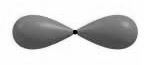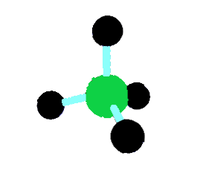GeeksforGeeks App
Open AppBrowser
Continue

## Related Articles

• CBSE Class 11 Chemistry Notes

# Valence-Shell Electron-Pair Repulsion (VSEPR) Theory

The Valence Shell Electron Repulsion (VSEPR) model can predict the structure of most molecules and polyatomic ions with a nonmetal central atom; it can also predict the structure of certain metal-centered molecules and polyatomic ions. VSEPR is based on Lewis electron dot structures, which can predict the geometry of each atom in a molecule when used combined. Lewis structures alone can only predict connectivity, but VSEPR and Lewis structures together can predict the geometry of each atom in a molecule.

VSEPR theory is based on the premise that pairs of electrons (in bonds and lone pairs) repel each other. “Groups” refer to electron pairs (both in bonds and lone pairs). Because electrons oppose each other electrostatically, the most stable electron group configuration (i.e., the one with the lowest energy) reduces repulsion.

The molecular structure with the lowest energy is created by arranging groups around the centre atom. To put it another way, the repulsion between groups around an atom promotes a geometry in which the groups are as far away as feasible. VSEPR is a simple model that successfully predicts the three-dimensional structures of a vast number of compounds, despite the fact that it does not account for the intricacies of orbital interactions that determine molecule shapes.

### Valence-Shell Electron-Pair Repulsion (VSEPR) Theory

Ronald Nyholm and Ronald Gillespie are the key creators of the VSEPR theory. To honour these chemists, this hypothesis is sometimes known as the Gillespie-Nyholm theory. The VSEPR theory states that the repulsion between two electrons is caused by the Pauli exclusion principle, which is more important than electrostatic repulsion in determining molecule shape.

The Valence Shell Electron Pair Repulsion Theory, abbreviated as VSEPR theory, is founded on the idea that all atoms have a repulsion between pairs of valence electrons, and that atoms will always seek to arrange themselves in a way that minimises this electron pair repulsion. The geometry of the final molecule is determined by the arrangement of the atoms.

The table below shows the various geometries that molecules can adopt in accordance with the VSEPR theory.

### Postulates of VSEPR Theory

1. In polyatomic molecules (molecules composed of three or more atoms), one of the constituent atoms is designated as the central atom, to which all other atoms in the molecule are attached.
2. The total amount of valence shell electron pairs determines the molecule’s structure.
3. Electron pairs have a tendency to organise themselves in such a way that their electron-electron repulsion is minimised while their distance is increased.
4. The valence shell can be visualised as a sphere with electron pairs clustered on its surface to reduce the distance between them.
5. If the core atom of the molecule is surrounded by electron bond pairs, an asymmetrically structured molecule can be expected.
6. If the centre atom is surrounded by both lone pairs and bond pairs of electrons, the molecule will be deformed.
7. The VSEPR theory can be applied to any molecule’s resonance structure.
8. The repulsion is largest between two lone pairs and smallest between two bond pairs.
9. If electron pairs around the core atom get too close, they will reject each other. As a result, the energy of the molecules increases.
10. If the electron pairs are separated by a large distance, the repulsions between them are reduced, and the energy of the molecule is reduced.

### Limitations of VSEPR Theory

1. This theory fails to account for isoelectronic species (i.e. elements having the same number of electrons). Despite having the same number of electrons, the shapes of the species can differ.
2. The VSEPR hypothesis does not throw any light on transition metal complexes. This theory cannot accurately describe the structure of several such molecules. This is because the VSEPR hypothesis does not account for the related sizes of the substituent groups and the inactive lone pairs.
3. Another drawback of VSEPR theory is that it predicts that halides of group 2 elements will have a linear structure whereas in fact they have a bent structure.

### Predicting the Shapes of Molecules

1. As the centre atom, the least electronegative atom must be chosen (since this atom has the highest ability to share its electrons with the other atoms belonging to the molecule).
2. The total number of electrons in the core atom’s outermost shell must be counted.
3. It is necessary to count the total number of electrons belonging to other atoms and utilise them in bonding with the centre atom.
4. These two numbers must be summed to yield the valence shell electron pair number, abbreviated as the VSEP number.

### Shapes

Linear Shape

There are two sites in the valence shell of the central atom in this sort of molecule. They should be organised in such a way that repulsion is reduced (pointing in the opposite direction). BeF2 is an example.Linear Shape

Trigonal Planar Shape

Three molecules are connected to a central atom in this sort of molecule. They are placed in such a way that electron repulsion between them is reduced (toward the corners of an equilateral triangle). BF3 is an example.Trigonal Planar Shape

Tetrahedral Shape

Atoms in two-dimensional molecules lie in the same plane, and if we apply these criteria to methane, we get a square planar geometry with a bond angle of 90 degrees between H-C-H. When all of these prerequisites for a three-dimensional molecule are met, we get a tetrahedral molecule in CH4.Tetrahedral Shape

Trigonal Bipyramid Shape

Let’s look at PF5 as an example. Repulsion can be reduced by distributing electrons evenly towards the corners of a trigonal pyramid. Three places along the molecule’s equator are found in trigonal bipyramids. The two places are parallel to each other on an axis perpendicular to the equatorial plane.Trigonal Bipyramidal Shape

### Predicting the Shapes of Molecules

The degree of the repulsion between a lone pair and an electron bond pair is intermediate between the repulsion between two lone pairs and two bond pairs. The repulsion order of electron pairs is

Lone Pair- lone pair > Lone Pair- bond- pair > Bond Pair- bond pair.

1. Total number of electron pairs surrounding the centre atom = 1/2 * (number of central atom valence electrons + number of atoms linked to central atom by single bonds).
2. For negative ions, add the number of electrons equal to the ions’ negative charge units to the central atom’s valence electrons. Subtract from the valence electrons of the central atom the number of electrons equal to the units of positive charge on the ion for positive ions.
3. Bond pair count = a total number of atoms linked to the centre atom via single bonds.
4. The number of lone pairs = a Total number of electrons – No of shared pair.
5. The electron pairs surrounding the core atom reject each other and migrate so far apart that there are no stronger repulsions between them. As a result, the molecule has the least amount of energy and the most stability.
6. A molecule with only two atoms always has a linear form.
7. When there are three or more atoms in a molecule, one of them is called the central atom, and the other atoms are connected to the central atom.
8. If the central atom is attached to comparable atoms and is surrounded by only electron bond pairs, the repulsions between them are similar, and the molecule’s form is symmetrical, and the molecule is said to have regular geometry.
9. The repulsion between them is the same if the core atom is linked to other atoms or is surrounded by a bond pair as well as a lone pair of electrons. As a result, the molecule’s form has an uneven or distorted geometry.
10. The molecule’s exact shape is determined by the total number of electron pairs present surrounding the core atom.

### Sample Questions

Question 1: What is the VSEPR Theory’s premise?

Because of the repulsion that exists between electron pairs in the valence shell, the atoms organise themselves in such a way that this repulsion is minimised. This has an immediate impact on the shape of the molecule generated by the atom.

Question 2: What would be the shape of the molecule if the VSEP number is 5?

The molecule’s structure would be trigonal bipyramidal.

Question 3: What are the advantages of the VSEPR theory?

This theory can be used to properly anticipate the shapes of molecules in a wide range of compounds. Understanding the processes of a molecule becomes easier once the geometry of the molecule is understood.

Question 4: What is the VSEPR number?